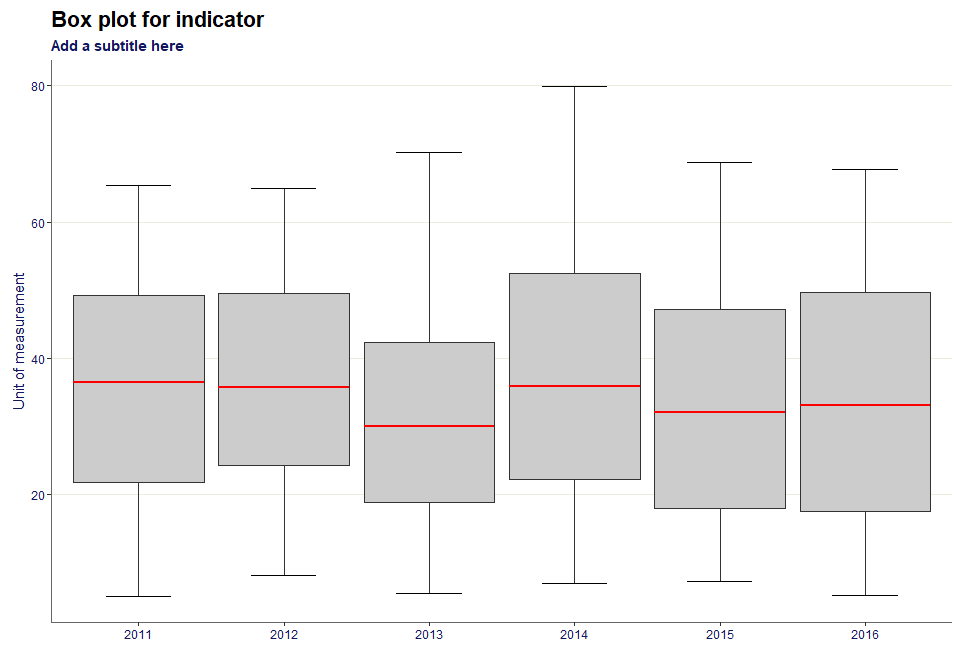# Producing charts from Fingertips

## Introduction

This vignette demonstrates how to create some of the charts available in this package. All of the names of the functions match the name for the chart on Fingertips.

### A note on ordering within the charts

The charts produced, generally, order the data alphabetically. In the static map section and the compare areas, there are worked examples of reordering the factor levels so they are presented in the required order in the legends.

## Libraries

library(fingertipscharts)
library(dplyr)
library(tidyr)

## Overview

This function plots the Overview view (also known as the Tartan Rug). First, let’s get some data:

region <- "PAC19"
top_names <- c("C001", region)
dfdom <- create_test_data() %>%
filter((AreaCode %in% top_names |
ParentAreaCode == region)) %>%
mutate(Value = round(Value, 1))

This is how the overview() function works:

p <- overview(data = dfdom,
area = AreaCode,
indicator = IndicatorName,
value = Value,
fill = Significance,
timeperiod = Timeperiod,
top_areas = top_names, wrap_length = 40,
value_label_size = 0.7)
p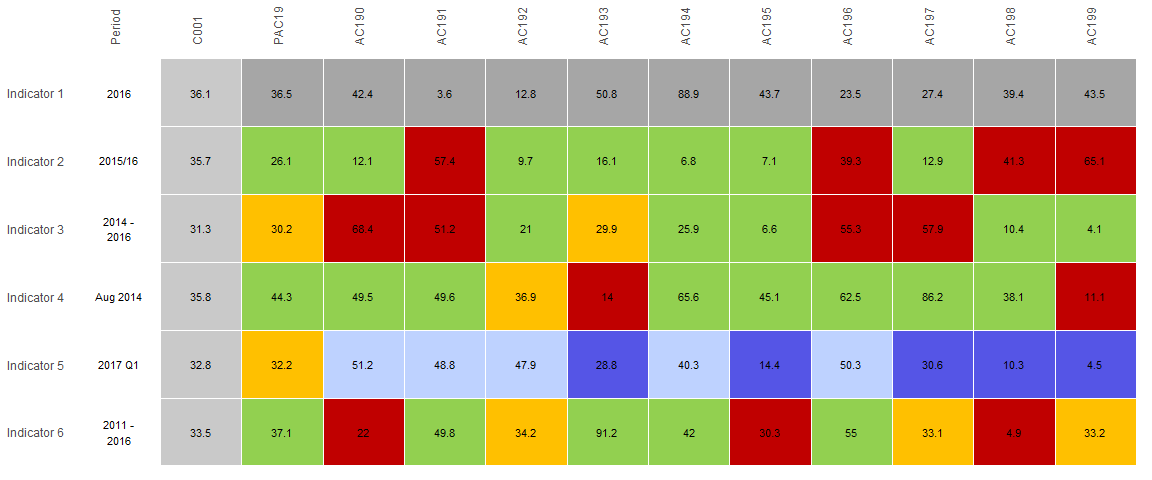## Compare indicators

Here is a function that helps produce a scatter plot as displayed on Fingertips. First the data needs to be changed to the correct format. The two variables that will be plotted need to be in separate fields. The spread() function from the tidyr package demonstrates how to do this below:

df <- create_test_data() %>%
filter(IndicatorName %in% c("Indicator 1", "Indicator 2")) %>%
select(IndicatorName, AreaCode, Value) %>%
mutate(IndicatorName = gsub(" ", "", IndicatorName)) %>%
pivot_wider(names_from = IndicatorName,
values_from = Value)

This is how the compare_indicators() function works:

p <- compare_indicators(data = df,
x = Indicator1,
y = Indicator2,
xlab = "This is indicator 1",
ylab = "This is indicator 2",
highlight = c("C001", "AC101"),
area = AreaCode,
p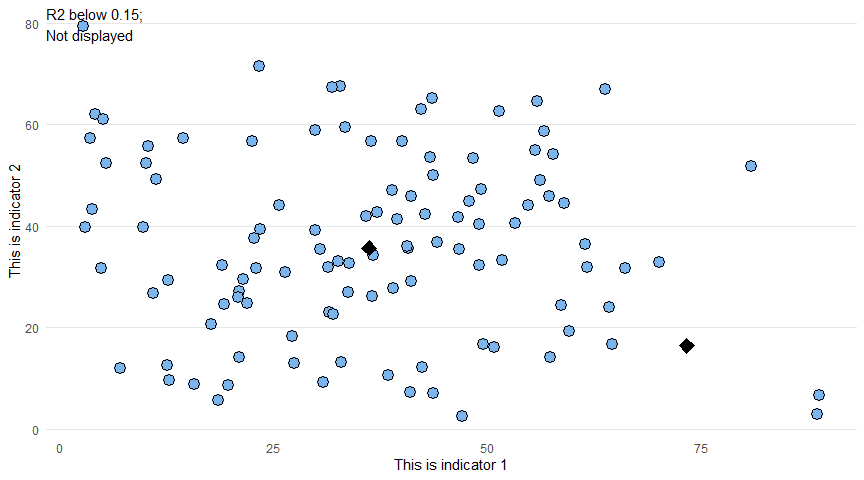## Maps

Here the package provides both interactive and static maps.

The shape files need to be sources from the ONS Geography Portal. To do this, click on the API button here, using Counties and Unitary Authorities as an example, and then use the GeoJSON address as the string input to the ons_api parameter.

ons_api <- "https://opendata.arcgis.com/datasets/687f346f5023410ba86615655ff33ca9_4.geojson"

### Static

Note, before plotting the chart, the levels of the field that is determining the fill of the polygons need to be reordered, as if they aren’t, they will be ordered alphabetically in the legend:

ordered_levels <- c("Better",
"Similar",
"Worse",
"Not compared")
df <- mapdata %>%
mutate(Significance =
factor(Significance,
levels = ordered_levels))

The default map type is static:

p <- fingertipscharts::map(data = df,
ons_api = ons_api,
area_code = AreaCode,
fill = Significance,
title = "Map title",
subtitle = "Map subtitle",
p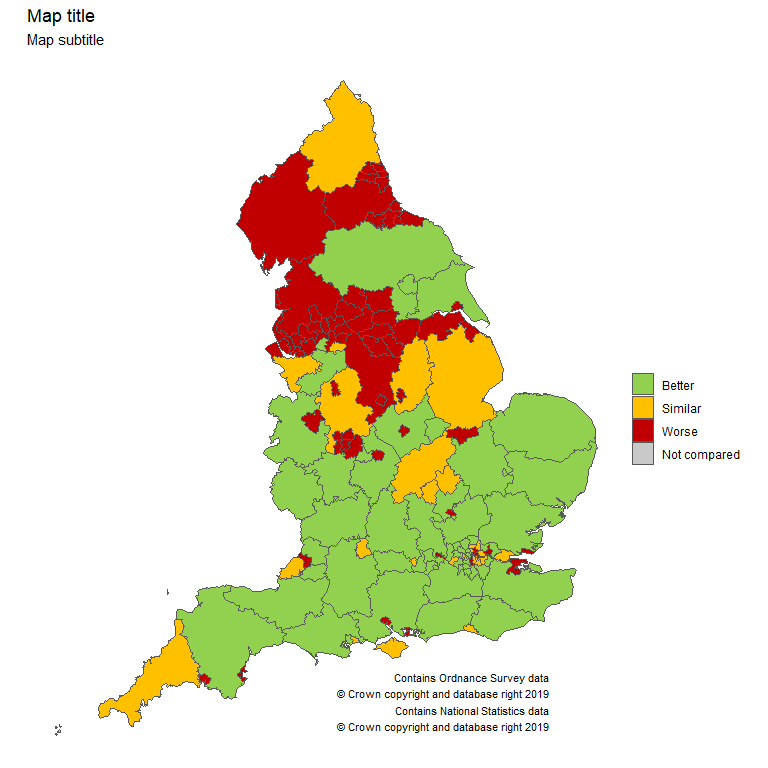### Interactive

It is also possible to have interactive maps. Note, for this to work the value parameter must be included and subtitle doesn’t work.

p <- map(df,
ons_api = ons_api,
area_code = AreaCode,
fill = Significance,
type = "interactive",
value = Value,
name_for_label = AreaName,
title = "Map title<br>with a line break")
p

## Compare areas

This code gets the latest year of data for IndicatorID 90316 filtered for local authorities whose parent is South East region and also the areas England and South East region:

region <- "PAC10"
top_names <- c("C001", region)
df <- create_test_data() %>%
filter(IndicatorName == "Indicator 3",
(ParentAreaCode == region |
AreaCode %in% top_names))

Note, before plotting the chart, the levels of the field that is determining the fill of the polygons need to be reordered, as if they aren’t, they will be ordered alphabetically in the legend:

ordered_levels <- c("Better",
"Similar",
"Worse",
"Not compared")
df <- df %>%
mutate(Significance =
factor(Significance,
levels = ordered_levels))

The compare_areas function makes plotting the compare areas chart straight forward.

p <- compare_areas(df,
AreaCode,
Value,
fill = Significance,
lowerci = LCI,
upperci = UCI,
order = "desc",
top_areas = top_names,
title = unique(df\$IndicatorName))
p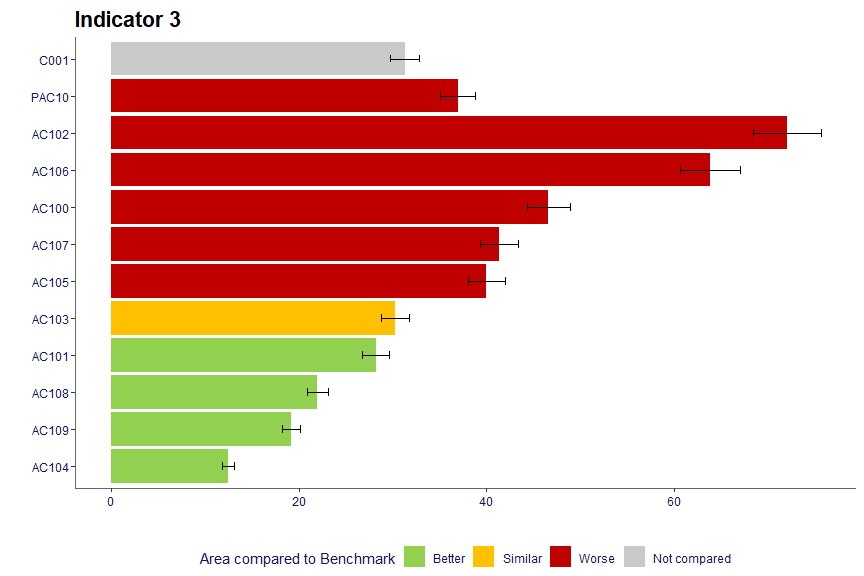## Area profiles

The area profiles image is created using the area_profiles() function. The argument rank = TRUE needs to be included as this returns a polarity field, which is needed for the spine chart:

dfspine <- create_test_data()
p <- area_profiles(dfspine,
value = Value,
count = Count,
area_code = AreaCode,
local_area_code = "AC110",
indicator = IndicatorName,
timeperiod = Timeperiod,
polarity = Polarity,
significance = Significance,
area_type = AreaType,
cols = "fingertips",
median_line_area_code = "C001",
comparator_area_code = "PAC11",
datatable = TRUE,
header_positions = c(-1, -0.63, -0.48, -0.36, -0.21, -0.05, 1.08),
relative_domain_text_size = 0.75,
relative_text_size = 1.2,
bar_width = 0.68)
p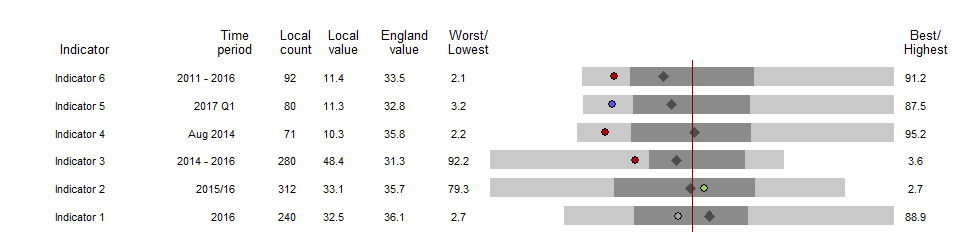## Population

This function provides the ability to draw the traditional population pyramid. First, the population data is extracted using fingertipsR package. Also, note that the ordering of the age bands are defined prior to the data being provided to the population() function:

set.seed(1234)
agelevels <- c("0-4", "5-9","10-14","15-19",
"20-24","25-29","30-34",
"35-39","40-44","45-49",
"50-54","55-59","60-64",
"65-69","70-74","75-79",
"80-84","85-89","90+")
local_males <- dnorm(seq(1, 19), mean = 5, sd = 5) * 1e5
local_females <- dnorm(seq(1, 19), mean = 4, sd = 5) * 1e5
region_males <- dnorm(seq(1, 19), mean = 5, sd = 7) * 1e5
region_females <- dnorm(seq(1, 19), mean = 4.5, sd = 6.5) * 1e5
country_males <- dnorm(seq(1, 19), mean = 4, sd = 3) * 1e5
country_females <- dnorm(seq(1, 19), mean = 5, sd = 3) * 1e5

pops <- data.frame(Value = c(local_males,
local_females,
region_males,
region_females,
country_males,
country_females),
AreaName = c(rep("Local", 38),
rep("Region", 38),
rep("Country", 38)),
Sex = rep(c(rep("Male", 19),
rep("Female", 19)),
times = 3),
stringsAsFactors = FALSE) %>%
mutate(Age = factor(rep(agelevels, 6),
levels = agelevels))

This is how the function works:

p <- population(pops,
value = Value,
sex = Sex,
age = Age,
area = AreaName,
area_name = "Local",
comparator_1 = "Country",
comparator_2 = "Region",
title = "Age Profile",
subtitle = "Area population for this time period",
xlab = "% of total population")
p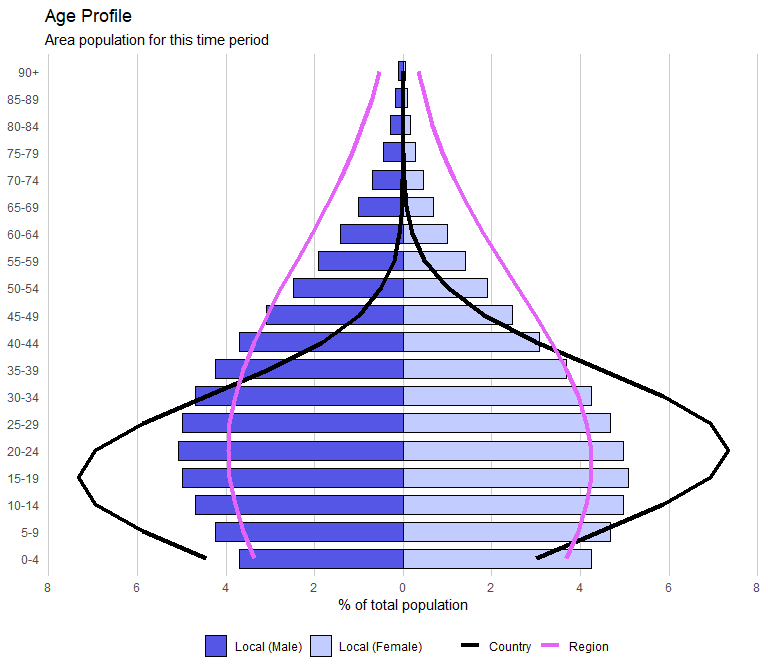## Box plots

Box plots can be found on some profiles. First the data is extracted using the fingertipsR package:

df <- create_test_data() %>%
arrange(IndicatorName) %>%
mutate(Timeperiod = rep(c("2011", "2012", "2013", "2014", "2015", "2016"),
each = 111))

This is how the function works. Note, the levels of the factor for the timeperiod parameter may need to be reordered, as this determines the order along the x-axis:

p <- box_plots(df,
timeperiod = Timeperiod,
value = Value,
title = "Box plot for indicator",
subtitle = "Add a subtitle here",
ylab = "Unit of measurement")
p